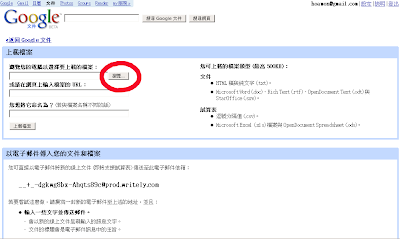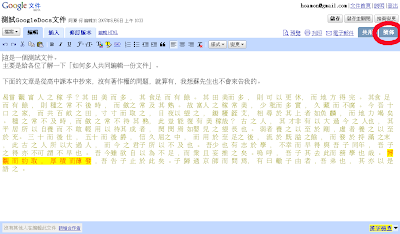## 2007年6月16日 星期六

### gnome桌面的「啟動」

skype 是 /usr/bin/skype
stardict 是 /usr/bin/stardict

## 2007年6月8日 星期五

To see is to believe(百聞不如一見)。

## 2007年6月6日 星期三

### 誰說Vista慢的!

Vista install in 2 minutes(兩分鐘內安裝 Windows Vista)

### CMClass: GA 演算法

f(x)= (x1/x2)* (sin(x2))^2 + (x2-x1)* cos(x1+10)
where
10<= x1 <= 30
30<= x2 <= 50

x1, x2 = any real value
try to find the minimum f(x)

` 1 def ConvVar(chromosom):     #轉換基因組到決策變數 2     variables = [] 3     variables.append((int(chromosom*3) + 1)*10) 4     variables += int(chromosom*10) 5     variables += int(chromosom*10)*0.1 6     variables += int(chromosom*10)*0.01 7     variables += int(chromosom*10)*0.001 8  9     variables.append((int(chromosom*3) + 3)*10)10     variables += int(chromosom*10)11     variables += int(chromosom*10)*0.112     variables += int(chromosom*10)*0.0113     variables += int(chromosom*10)*0.00114 15     return variables16 17 from math import sin, cos18 def FUNC(variables):        #定義目標函數19     (x1, x2) = variables20     if x1 > 30 or x1 < 10: return float('infinity')21     if x2 > 50 or x2 < 30: return float('infinity')22     sum = (x1/x2) * (sin(x2)) * (sin(x2)) + (x2-x1) * cos(x1+10)23     return sum`

### 我不太愛收到 .doc / .odt / .xls / .ods 的檔案

1. 內容有趣
2. 工作上需要，需要撰寫一份圖文並茂的文章。